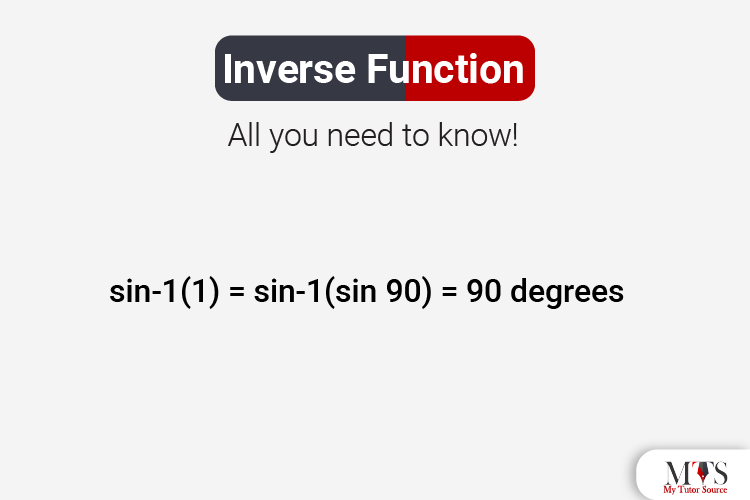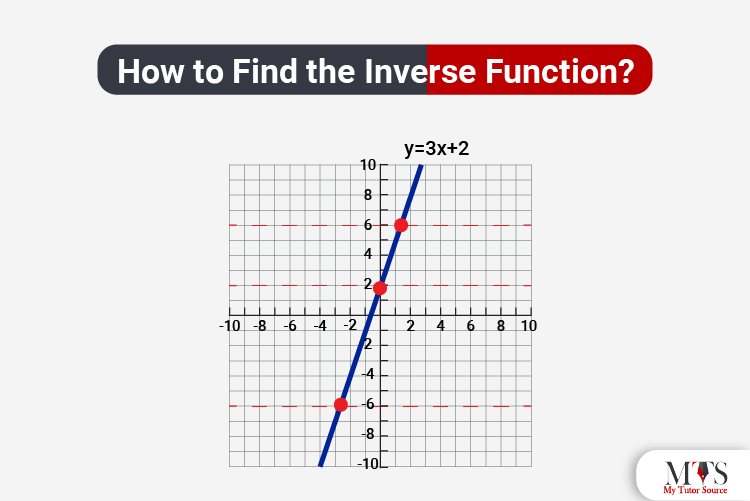# Inverse Function: All you need to know!Mathematics is full of practical concepts; they may be hard to understand, but super interesting when you get the hang of them. One of those practical concepts is known as inverse function - and we are here to make this concept easy and interesting for you.

As the name implies, inverse functions always go the other way! In simple words, an inverse function is the opposite of a function. In this post, we are going to learn more about the inverse function, its types, how to find it, and solved examples for better understanding. Keep Reading!

## What is Inverse Function?

The inverse function, also known as an anti function, can inverse itself into another function. In simpler words, if a function is 'f', and it takes to x to y, then the inverse function will take to y to x. Also, if a function is represented as F or f, then the inverse function will be represented as f-1 or F-1. However, keep in mind that the -1 here is not a reciprocal or an exponent. The scientific definition of a function is:
"The inverse function returns the original value for which a function gave the output."
A function can perform operations, accept values, and generates outputs on that values. While on the other hand, an inverse function operates, agrees with the resultant, and reaches back to the original function.

In trigonometry, we use the inverse sine function to find the measurement of the angle for which the sine function generated the value. For example,

sin-1(1) = sin-1(sin 90) = 90 degrees
Therefore, the sin 90 degrees will be equal to 1.

## How to Find the Inverse Function?

The general method to calculate and find the inverse function is by swapping the sides of x and y. Moreover, to find the inverse function, we have to make sure that the original function is a one-to-one function. The functions whose second elements will always correspond to the first values are called one-to-one functions. To check if a function is one-to-one or not, you can apply the horizontal line test.Here are a few steps to help you find inverse functions conveniently. In this example, we suppose a function, f(x) = ax + b. Now, we will find the inverse of this function by the following steps:
1. The given function composition is f(x) = ax + b. We will replace f(x) = y to get y = ax + b.
2. Then, we will interchange the y with x and x with y in the function y = ax + b to obtain x = ay + b.
3. Now, we solve the expression x = ay + b, and get (x - b)/a.
4. Now finally, we will replace y = f-1(x), and we will have f-1(x) = (x - b)/a.

## Inverse Function Graph

The graph of an inverse function always shows two things: the function, and the inverse of the function over the line y = x. This line goes through the origin and has a slope value of 1 in the graph. Hence, you will represent it as:
y = f-1(x)
which will be equal to,
x = f(y)
This relation is kind of identical to the y = f(x) one. F is the same, but the roles of x and y are reversed. Hence, if we wish to draw the graph of f-1, then we have to switch the positions of x and y.

## Types of Inverse Function

There are different types of inverse functions including rational functions, trigonometric functions, log functions, etc. We will go through the most common types in detail now. But first, let's briefly go through the inverses of some of the most common functions:

 Function Inverse of the Function Comment + – × / Don’t divide by 0 1/x 1/y x and y not equal to 0 x2 √y x and y ≥ 0 xn y1/n n is not equal to 0 ex ln(y) y > 0 ax log a(y) y and a > 0 Sin (x) Sin-1 (y) – π/2 to + π/2 Cos (x) Cos-1 (y) 0 to π Tan (x) Tan-1 (y) – π/2 to + π/2

### Inverse Trigonometric Functions

Inverse trigonometric functions, also known as arc functions, help in producing the length of the arc. This length is further used to obtain the particular value on which the function is applied. There are a total of six inverse trigonometric functions:
1. Arcsine (sin-1)
2. Arccosine (cos-1)
3. Arctangent (tan-1)
4. Arc secant (sec-1)
5. Arc cosecant (cosec-1)
6. Arc cotangent (cot-1)

### Inverse Rational Function

A rational function is the function of form, f(x) = P(x)/Q(x) where Q(x) ≠ 0. Here are a few steps with a supposed example to find the inverse of a rational function:

Step 1: Replace f(x) = y.
Step 2: Interchange x and y.
Step 3: Start solving y in terms of x.
Step 4: Replace y with f-1 (x).
Step 5: You have the inverse of the function.

### Inverse Hyperbolic Function

The methodology and concept of these are somewhat similar to inverse trigonometric functions. In inverse hyperbolic function, you have to find the inverses of the hyperbolic functions. There are a total of 6 types of inverse hyperbolic functions:
1. sinh-1
2. cosh-1
3. tanh-1
4. csch-1
5. coth-1
6. sech-1

## Inverse Functions Examples

Now that we have gone through the main concept of inverse functions, it is time to go through some solved examples for a better and more effective understanding. Read on!

### Example 1:

We have a function function f(x) = ln(x – 2). What will be its inverse?
Solution:
First of all, we will replace f(x) with y.
So, y = ln(x – 2)
Now, we replace the equation in an exponential way, x – 2 = ey
Now, solving for x,
x = 2 + ey
Now, we will replace x with y
thus, f-1(x) = y = 2 + ey

### Example 2:

Solve: f(x) = 2x + 3, at x = 4
Solution:
f(4) = 2 × 4 + 3
f(4) = 11
Now, we will apply for reverse on 11.
f-1(11) = (11 – 3) / 2
f-1(11) = 4
Here we get 4 again.
Therefore, f-1(f(4)) = 4
So, when we apply function f and its reverse f-1 gives the original value back again, i.e,
f-1(f(x)) = x.

## Conclusion

Inverse functions may sound difficult, but trust me; the concept is super straightforward. We tried to explain everything in detail in this post. However, if you still have any questions, you can always hire a highly qualified private tutor from our website. Our professional tutors will clear away all your confusions and doubts.

So, what are you waiting for? Your tutor is just a click away!

## Frequently Asked Questions

What is the inverse function?
An inverse function is a function that can reverse itself into another function. It is also known as an anti-function.
What is a bijective function?
A function is known as a bijective function, if a function f: A → B satisfies both the injective (one-to-one function) and surjective function (onto function) properties.
What is an identity function?
The identity function is the function whose value is always equal to the value of an independent variable.
What is an injective function?
An injective function, also known as a one-to-one function, is a function that combines distinct elements with distinct elements.
What is the inverse of 1/x?
Let f(x) = 1/x = y
Then inverse of f(x) will be f-1(y).
f-1(y) = 1/x

### Find Top Tutors in Your AreaWith over 3 years of experience in teaching, Chloe is very deeply connected with the topics that talk about the educational and general aspects of a student's life. Her writing has been very helpful for students to gain a better understanding of their academics and personal well-being. I’m also open to any suggestions that you might have! Please reach out to me at chloedaniel402 [at] gmail.com